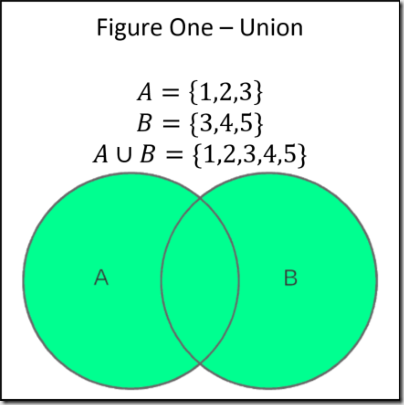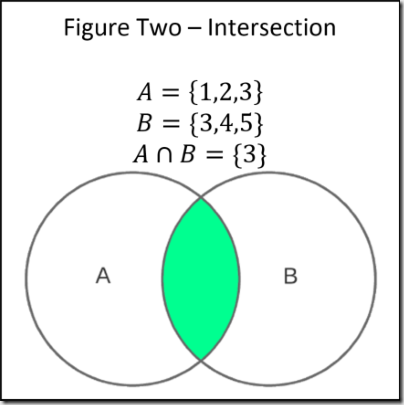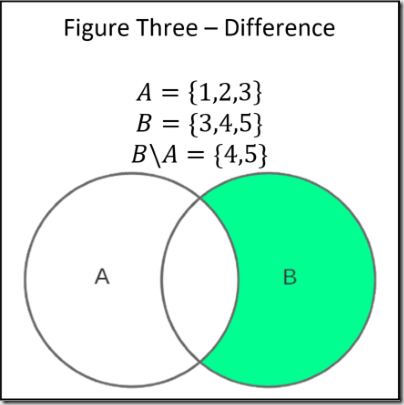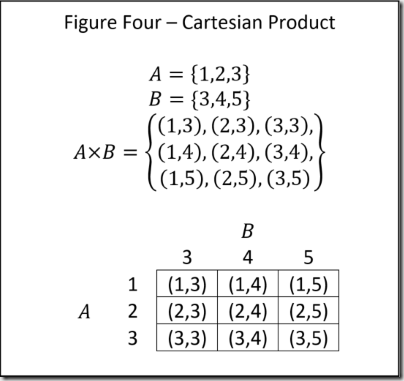# Just Enough Set Theory - When Sets Collide (Part 3 of 3)

Welcome to the final installment of this three-part series on set theory. The first piece, Set Theory Defined, detailed requisite foundational knowledge. The second article, Set Operations, outlined some beneficial set algorithms. This post develops the concepts laid out in the first two; therefore, it is highly recommended that readers begin there.

Individual sets have many useful properties; however, preforming operations on multiple sets provides even greater utility. This piece outlines four such operations. Each operation provides a concise means for addressing common programming problems that virtually all software professionals encounter. There is a brief description of each from a mathematical perspective followed by JavaScript (ES6) code excerpts demonstrating how to apply theory to real world scenarios.

NOTE: All code samples are written in ES6 and are therefore not likely to execute directly in a browser. The best option is to use Node or transpile the excerpts using either Babel or TypeScript. The working code is available on GitHub along with execution instructions.

## Union

The union of two sets is a set containing the distinct elements from both sets. $\cup$ is the mathematical symbol for a union and the union of sets $A$ and $B$ is denoted as $A \cup B$ . An expanded way of representing the union relationship is $\{x| x \in A \vee x \in B\}$ , which means every element contained in $A$ OR ($∨$) $B$. Figure One – Union depicts two sets with three elements each. The union is a set with five elements because one item, three, is shared and union returns distinct values. The Venn diagram shows the relationship graphically.Generating the union of two sets is quite easy in ES6 as the code below illustrates.

const A = new Set([1, 2, 3]);
const B = new Set([3, 4, 5]);
const union = new Set([...A, ...B]);
// union = [1,2,3,4,5];


The astute reader will notice that there’s some legerdemain afoot. The code above uses the ES6 Set data structure instead of standard JavaScript arrays. Set holds only unique elements by ignoring add operations for new values that match existing ones. The algorithm is as easy as concatenating the two sets without the concern of distinct elements. If the code was using standard arrays, there would have to be logic to remove duplicated items. Luckily, converting between sets and arrays is virtually effortless.

const setDataStructure = new Set([1, 2, 3]);
const arrayDataStrcture = Array.from(setDataStructure);


The problem with the code above is that it’s a rare requirement to union sets containing primitive values. Software engineering is seldom that straightforward. A more realistic scenario is calculating the union between two sets of complex objects where equality becomes problematic. Unlike primitive variables, objects with identical values are not equal because they compare by reference. This abrogates the Set trick from earlier. Suppose the requirement is to compute all bug reports currently in process across two teams and it’s possible that both teams are working on the same bugs simultaneously. The code below demonstrates a solution by first concatenating the two sets and then removing duplicates using the filter method introduced in the last article. Notice the only equality check is via the Id. Obviously, this won’t work for every scenario and depending on the size of the sets and performance requirements it is possible to write generic deep equality methods (or use a library like underscore).

const teamABugs = [
{ id: 1, name: "Screen Explodes" },
{ id: 2, name: "Keyboard Burts into Flames" },
{ id: 3, name: "Submit button off by 1 pixel" }];
const teamBBugs = [
{ id: 5, name: "Randomly Dials Russian Hackers" },
{ id: 6, name: "Publishes CC info to the www" },
{ id: 3, name: "Submit button off by 1 pixel" }];

const union = [...teamABugs, ...teamBBugs]
.filter((x, index, array) => array.findIndex(y => y.id == x.id) == index);


## Intersection

The intersection of two sets is a set containing distinct shared elements. $A \cap B$ is the mathematical representation of a union and the expanded notation is $\{x|x \in A \wedge x \in B \}$. Stated differently, the intersection of set $A$ AND ($\wedge$) $B$ is every element contained in $A$ AND $B$. Figure Two – Intersection depicts the relationship showing the union of $A$ and $B$ to be a singleton set containing only the number three. Once again, the Venn diagram portrays the relationship.Much like union, finding the intersection of two sets using the Set data structure and primitive types is easy. The code below shows how it’s a matter of using the filter method to check to see if an item is also stored in the other set.

const A = new Set([1, 2, 3]);
const B = new Set([3, 4, 5]);
const intersect = [...A].filter(x => B.has(x));
// intersect = ;


The code above is a bit fanciful. Consider instead a role protected resource. Possessing any one of many roles allows users to access said resource. Users each have a set of associated roles. There are a few different ways to achieve this, but finding the intersection between the user’s roles and the resource’s required roles is the most manageable. See the code below.

const resourceRoles = [
{ id: 1, name: "Administrator" },
{ id: 2, name: "Super User" }];
const user =  { id: 314, name: "Edsger Dijkstra", roles: [
{ id: 1, name: "Administrator" },
{ id: 2, name: "User" }] }

const hasAccess = resourceRoles
.filter(x => user.roles.find(y => y.name == x.name)).length > 0;


All of the caveats about equality described in the Union section also apply here. It’s something programmers need to be cognizant of.

## Difference

The difference of two sets is sometimes known as the relative complement; both nomenclatures are interchangeable. The concept is simple, the difference is a set made up of the items that are left over after removing the intersection of another set. Otherwise stated, all of the items in set $B$ that do not exist in set $A$. Mathematically, this is represented as $\{x|x \in B \wedge x \notin A\}$ or the shortened version which is $B \setminus A$. Figure Three – Difference shows the difference between $B$ and $A$ to be a set containing four and five. Just as above, there is a representative Venn diagram.As an aside, there is also an absolute compliment which is somewhat similar; however, it is outside the scope of this article.

Finding the difference of sets is almost identical to finding the intersection as the code below demonstrates. The only variation is that the predicate passed to the filter method is negated.

const A = new Set([1, 2, 3]);
const B = new Set([3, 4, 5]);
const difference = [...B].filter(x => !A.has(x));
// difference = [4,5];


Again, a more realistic example is in order. Image that there is a set of actions that must be completed and a set of actions a user has completed. Finding the difference is an easy way to determine if all required actions are complete.

const requiredActions = [
{ id: 1, name: "Electronic Signing" },
{ id: 2, name: "Submission Form" },
{ id: 3, name: "Payment" }];
const userActions = [
{ id: 1, name: "Electronic Signing" },
{ id: 2, name: "Submission Form" }];

const complete = requiredActions
.filter(x => !userActions.find(y => y.name == x.name)).length === 0;
// complete = false


## Cartesian Product

The Cartesian product of two sets is a set of ordered pairs that contain all possible combinations of elements in the two sets. The mathematical representation is $A \times B$. The expanded notation is $\{(a,b)|a \in A \wedge b \in B\}$ which means an ordered pair consisting of every element in $A$ AND ($\wedge$) every element in $B$. Figure Four – Cartesian Product demonstrates the concept. As a matter of importance, unlike standard products, the Cartesian product is not commutative. Stated mathematically, $A \times B \ne B \times A$. Switching the order of statement will change the order of the pairs.The Cartesian product is useful for combinatorics problems. A common example is simulating a deck of cards. Instead of specifying all the cards explicitly in code, it’s easier to define the suits and values as two separate sets and then take the Cartesian product to get the entire deck. See the code below.

const suits = ['Diamond', 'Spade', 'Heart', 'Club'];
const values = ['Ace', '2', '3', '4', '5', '6', '7', '8', '9', '10', 'Jack', 'Queen', 'King'];

const cards = suits.reduce((acc, x) => [...acc, ...values.map(y => [x, y])], []);
// Alternatively, it’s possible to return the ordered pair as an object instead of an array
// const cards = suits.reduce((acc, x) => [...acc, ...values.map(y => { return { suit: x, value: y } })], []);


This code should be starting to look familiar because all the samples make heavy use of the map, reduce, and filter methods. Using ES6, these methods have great utility for mimicking mathematical set operations. Because the code above is similar to previous examples, it doesn’t require further explanation.

## Why Stop at Two?

Up to this point, all the exhibited set operations employ two sets. However, this is for the sake of brevity. Each operations can act on as many sets as required. For instance, $A \cup B \cup C$ is perfectly valid as is $A \times B \times C$. The enthused reader should solidify his/her learning by expanding each code sample to use additional sets.

## Real World Applications

This series demonstrated how set theory is applied to data structures and demonstrated some novel uses for set operations in order create efficient algorithms. However, this is only a meager representation of all the many and varied applications for software engineering. Relational databases make heavy use of set theory for defining data structure and constructing data queries. In fact, SQL is essentially a set notation. There are several instances in language theory and design where strings are realized as sets and set operations are performed on them. Another prolific use is in computer graphics where points on a plane are treated as sets. The list of applications is considerable. It’s a body of knowledge that no software professional should forsake.

## Conclusion

Thus concludes this three-part series on set theory. Hopefully, the reader has gained a high-level understanding as well as enough practical knowledge to apply the learning forthwith. The first article outlined the basics and introduced the concept of set mapping. Empty sets, cardinality, subsets, summation, and power sets were introduced in the second piece. Finally, this post presented operations involving more than one set including unions, intersections, differences, and Cartesian products. The method was to first introduce the ideas mathematically and then demonstrate how to apply them using ES6. These concepts should not be considered optional for software professionals because set theory is ubiquitous in computer science.

As always, thank you for reading and please feel free to contact me with questions. I’m also happy to create more in depth posts upon request.